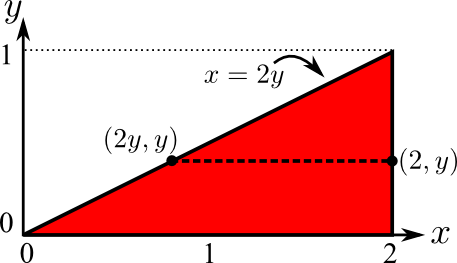# Math Insight

### Image: Double integral over triangular region, integrating y firstThe red region is a triangle over which we can compute a double integral. Since the domain is described as $0 \le y \le 1$ and $2y \le x \le 2$, it is set up to integrate with respect to $y$ first.

Image file: double_integral_over_triangle_y_first.png

Source image file: double_integral_over_triangle_y_first.svg
Source image type: Inkscape SVG